Do you like the article?
Share it with others -
post a link to it!
Use new possibilities of MetaTrader 5

# Graphical Interfaces X: Updates for the Rendered table and code optimization (build 10)

27 March 2017, 18:18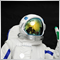0
2 647

### Introduction

The first article Graphical Interfaces I: Preparation of the Library Structure (Chapter 1) considers in detail what this library is for. You will find a list of articles with links at the end of each chapter. There, you can also download a complete version of the library at the current stage of development. The files must be located in the same directories as in the archive.

We continue to complement the rendered table (CCanvasTable) with new features. The following features will be added this time.

• Highlighting the table rows when hovered.
• The ability to add an array of icons for each cell and a method for switching them.
• The ability to set and modify the text in cell during the runtime.

In addition, the code and certain algorithms have been optimized to redraw the table faster.

### Relative coordinates of the cursor on the specified canvas

To eliminate the duplicate code in many classes and methods for calculating the relative coordinates on the canvas, the CMouse::RelativeX() and CMouse::RelativeY() methods have been added to the CMouse class for retrieving the coordinates. A reference to an object of the CRectCanvas type must be passed to these methods in order to calculate the relative coordinate with consideration of the current offset of the visible part of the canvas.

```//+------------------------------------------------------------------+
//| Class for getting the mouse parameters                           |
//+------------------------------------------------------------------+
class CMouse
{
public:
//--- Returns the relative coordinates of the mouse cursor from the passed canvas object
int               RelativeX(CRectCanvas &object);
int               RelativeY(CRectCanvas &object);
};
//+------------------------------------------------------------------+
//| Returns the relative X coordinate of the mouse cursor            |
//| from the passed canvas object                                    |
//+------------------------------------------------------------------+
int CMouse::RelativeX(CRectCanvas &object)
{
return(m_x-object.X()+(int)object.GetInteger(OBJPROP_XOFFSET));
}
//+------------------------------------------------------------------+
//| Returns the relative Y coordinate of the mouse cursor            |
//| from the passed canvas object                                    |
//+------------------------------------------------------------------+
int CMouse::RelativeY(CRectCanvas &object)
{
return(m_y-object.Y()+(int)object.GetInteger(OBJPROP_YOFFSET));
}
```

In further development of the library, these methods will be used to obtain the relative coordinates of all drawn controls.

### Changes in the structure of the table

To optimize the code execution of the rendered table as much as possible, it was necessary to slightly modify and supplement the table's structure of the CTOptions type, and add new structures which allow building multidimensional arrays. The task here is to make certain fragments of the table redraw based on previously calculated values. For example, these may be the coordinates of the table columns and rows borders.

For instance, calculating and storing the X coordinate of the column borders is only reasonable in the CCanvasTable::DrawGrid() method, which is used for drawing the grid and only when drawing the entire table. And when user selects a table row, the predetermined values can be used. The same applies to the highlighting of table rows when hovering (this will be discussed further in the article).

Create a separate structure (CTRowOptions) and declare an array of its instances to store the Y coordinates of the table rows, and possibly other row properties in the future. The Y coordinates of the rows are calculated in the CCanvasTable::DrawRows() method, designed for drawing the background of the rows. Since this method is called before drawing the grid, the CCanvasTable::DrawGrid() method uses the precalculated values from the CTRowOptions structure.

Create a separate structure of the CTCell type for storing the table cell properties. The array of instances in the CTRowOptions structure is declared with this type, as an array of table rows. This structure will store:

• Array of icons
• Arrays of icon sizes
• Index of the selected (displayed) icon in a cell
• Full text
• Shortened text
• Text color

Since each icon is an array of pixels, a separate structure (CTImage) with a dynamic array for storing them will be required. The code of these structures can be found in the listing below:

```class CCanvasTable : public CElement
{
private:
//--- Array of the icon pixels
struct CTImage { uint m_image_data[]; };
//--- Properties of the table cells
struct CTCell
{
CTImage           m_images[];       // Array of icons
uint              m_image_width[];  // Array of icon widths
uint              m_image_height[]; // Array of icon heights
int               m_selected_image; // Index of the selected (displayed) icon
string            m_full_text;      // Full text
string            m_short_text;     // Shortened text
color             m_text_color;     // Text color
};
//--- Array of rows and properties of the table columns
struct CTOptions
{
int               m_x;             // X coordinate of the left edge of the column
int               m_x2;            // X coordinate of the right edge of the column
int               m_width;         // Column width
ENUM_ALIGN_MODE   m_text_align;    // Text alignment mode in the column cells
int               m_text_x_offset; // Text offset
string            m_header_text;   // Column header text
CTCell            m_rows[];        // Array of the table rows
};
CTOptions         m_columns[];
//--- Array of the table row properties
struct CTRowOptions
{
int               m_y;  // Y coordinate of the top edge of the row
int               m_y2; // Y coordinate of the bottom edge of the row
};
CTRowOptions      m_rows[];
};
```

The appropriate changes have been made to all methods where these data types are used.

### Determining the range of rows in visible part

Since a table may have numerous rows, the search of the focus on a row followed by redrawing the table can significantly slow down the process. The same applies to selecting a row and adjusting the text length during changing the column width manually. In order to avoid lagging, it is necessary to determine the first and the last index in the visible part of the table and arrange a cycle to iterate within that range only. The CCanvasTable::VisibleTableIndexes() method has been implemented for this purpose. It first determines the boundaries of the visible part. The upper boundary is the offset of the visible part along the Y axis, and the lower boundary is defined as the upper + the size of the visible part along the Y axis.

It is now sufficient to divide the obtained boundary values by the row height defined in the table settings in order to determine the indexes of the top and bottom rows of the visible part. In case the range of the last table row has been exceeded, adjustment is performed at the end of the method.

```class CCanvasTable : public CElement
{
private:
//--- For determining the indexes of the visible part of the table
int               m_visible_table_from_index;
int               m_visible_table_to_index;
//---
private:
//--- Determining the indexes of the visible part of the table
void              VisibleTableIndexes(void);
};
//+------------------------------------------------------------------+
//| Constructor                                                      |
//+------------------------------------------------------------------+
CCanvasTable::CCanvasTable(void) : m_visible_table_from_index(WRONG_VALUE),
m_visible_table_to_index(WRONG_VALUE)
{
...
}
//+------------------------------------------------------------------+
//| Determining the indexes of the visible part of the table         |
//+------------------------------------------------------------------+
void CCanvasTable::VisibleTableIndexes(void)
{
//--- Determine the boundaries taking the offset of the visible part of the table into account
int yoffset1 =(int)m_table.GetInteger(OBJPROP_YOFFSET);
int yoffset2 =yoffset1+m_table_visible_y_size;
//--- Determine the first and the last indexes of the visible part of the table
m_visible_table_from_index =int(double(yoffset1/m_cell_y_size));
m_visible_table_to_index   =int(double(yoffset2/m_cell_y_size));
//--- Increase the lower index by one, if not out of range
m_visible_table_to_index=(m_visible_table_to_index+1>m_rows_total)? m_rows_total : m_visible_table_to_index+1;
}
```

The indexes will be determined in the CCanvasTable::DrawTable() method. This method can be passed an argument to specify that it is necessary to redraw only the visible part of the table. The default value of the argument is false, which indicates redrawing the entire table. The code listing below shows the shortened version of this method.

```//+------------------------------------------------------------------+
//| Draw table                                                       |
//+------------------------------------------------------------------+
void CCanvasTable::DrawTable(const bool only_visible=false)
{
//--- If not indicated to redraw only the visible part of the table
if(!only_visible)
{
//--- Set the row indexes of the entire table from the beginning to the end
m_visible_table_from_index =0;
m_visible_table_to_index   =m_rows_total;
}
//--- Get the row indexes of the visible part of the table
else
VisibleTableIndexes();
//--- Draw the background of the table rows
//--- Draw a selected row
//--- Draw grid
//--- Draw icon
//--- Draw text
//--- Display the latest drawn changes
//--- Update headers, if they are enabled
//--- Adjustment of the table relative to the scrollbars
}
```

A call to the CCanvasTable::VisibleTableIndexes() is also necessary in the method for determining the focus on the table rows:

```//+------------------------------------------------------------------+
//| Checking the focus on the table rows                             |
//+------------------------------------------------------------------+
int CCanvasTable::CheckRowFocus(void)
{
int item_index_focus=WRONG_VALUE;
//--- Get the relative Y coordinate below the mouse cursor
int y=m_mouse.RelativeY(m_table);
///--- Get the indexes of the local area of the table
VisibleTableIndexes();
//--- Search for focus
for(int i=m_visible_table_from_index; i<m_visible_table_to_index; i++)
{
//--- If the row focus changed
if(y>m_rows[i].m_y && y<=m_rows[i].m_y2)
{
item_index_focus=i;
break;
}
}
//--- Return the index of the row with the focus
return(item_index_focus);
}
```

### Icons in the table cells

Multiple icons can be assigned to each cell, which can be switched during the program runtime. Add fields and methods for setting the icon offsets from the top and left edges of the cell:

```class CCanvasTable : public CElement
{
private:
//--- Icon offsets from the cell edges
int               m_image_x_offset;
int               m_image_y_offset;
//---
public:
//--- Icon offsets from the cell edges
void              ImageXOffset(const int x_offset)     { m_image_x_offset=x_offset;       }
void              ImageYOffset(const int y_offset)     { m_image_y_offset=y_offset;       }
};
```

To assign icons to the specified cell, it is necessary to pass an array with their paths in the local directory of the terminal. Prior to this, they must be included in the MQL application as resources (#resource). The CCanvasTable::SetImages() method is designed for this purpose. Here, if an empty array is passed or array overrun is detected, the program leaves the method.

If the checks are passed, the arrays of the cell are resized. After that, a cycle uses the ::ResourceReadImage() method to read the icon content to a one-dimensional array, storing the color of each pixel into the array. The icon sizes are stored to the corresponding arrays. They will be needed to arrange the cycles for drawing the icons on the canvas. The first icon of the array will be selected in the cell by default.

```class CCanvasTable : public CElement
{
public:
//--- Set icons to the specified cell
void              SetImages(const uint column_index,const uint row_index,const string &bmp_file_path[]);
};
//+------------------------------------------------------------------+
//| Set icons to the specified cell                                  |
//+------------------------------------------------------------------+
void CCanvasTable::SetImages(const uint column_index,const uint row_index,const string &bmp_file_path[])
{
int total=0;
//--- Leave, if a zero-sized array was passed
if((total=CheckArraySize(bmp_file_path))==WRONG_VALUE)
return;
//--- Checking for exceeding the array range
if(!CheckOutOfRange(column_index,row_index))
return;
//--- Resize the arrays
::ArrayResize(m_columns[column_index].m_rows[row_index].m_images,total);
::ArrayResize(m_columns[column_index].m_rows[row_index].m_image_width,total);
::ArrayResize(m_columns[column_index].m_rows[row_index].m_image_height,total);
//---
for(int i=0; i<total; i++)
{
//--- The first icon of the array is selected by default
m_columns[column_index].m_rows[row_index].m_selected_image=0;
//--- Write the passed icon to the array and store its size
if(!ResourceReadImage(bmp_file_path[i],m_columns[column_index].m_rows[row_index].m_images[i].m_image_data,
m_columns[column_index].m_rows[row_index].m_image_width[i],
m_columns[column_index].m_rows[row_index].m_image_height[i]))
{
Print(__FUNCTION__," > error: ",GetLastError());
return;
}
}
}
```

To find out how many icons a particular cell has, use the CCanvasTable::ImagesTotal() method:

```class CCanvasTable : public CElement
{
public:
//--- Returns the total number of icons in the specified cell
int               ImagesTotal(const uint column_index,const uint row_index);
};
//+------------------------------------------------------------------+
//| Returns the total number of icons in the specified cell          |
//+------------------------------------------------------------------+
int CCanvasTable::ImagesTotal(const uint column_index,const uint row_index)
{
//--- Checking for exceeding the array range
if(!CheckOutOfRange(column_index,row_index))
return(WRONG_VALUE);
//--- Return the size of the icons array
return(::ArraySize(m_columns[column_index].m_rows[row_index].m_images));
}
```

Now consider the methods that will be used for drawing the icons. First of all, a new CColors::BlendColors() method has been added to the CColors class, which allows correctly blending the upper and lower colors taking into account the transparency of the overlay icon. As well as an auxiliary CColors::GetA() method for getting the transparency value of the passed color.

In the CColors::BlendColors() method, the passed colors are first split into the RGB components, and the alpha channel is extracted from the top color. The alpha channel is converted to a value between zero and one. If the passed color does not contain transparency, blending is not performed. In case there is transparency, then each component of the two passed colors is blended taking into account the transparency of the top color. After that the values of the obtained components are adjusted, in case they are out of range (255).

```//+------------------------------------------------------------------+
//| Class for working with color                                     |
//+------------------------------------------------------------------+
class CColors
{
public:
double            GetA(const color aColor);
color             BlendColors(const uint lower_color,const uint upper_color);
};
//+------------------------------------------------------------------+
//| Getting the A component value                                    |
//+------------------------------------------------------------------+
double CColors::GetA(const color aColor)
{
return(double(uchar((aColor)>>24)));
}
//+------------------------------------------------------------------+
//| Blending two colors considering the transparency of color on top |
//+------------------------------------------------------------------+
color CColors::BlendColors(const uint lower_color,const uint upper_color)
{
double r1=0,g1=0,b1=0;
double r2=0,g2=0,b2=0,alpha=0;
double r3=0,g3=0,b3=0;
//--- Convert the colors in ARGB format
uint pixel_color=::ColorToARGB(upper_color);
//--- Get the components of the lower and upper colors
ColorToRGB(lower_color,r1,g1,b1);
ColorToRGB(pixel_color,r2,g2,b2);
//--- Get the transparency percentage from 0.00 to 1.00
alpha=GetA(upper_color)/255.0;
//--- If there is transparency
if(alpha<1.0)
{
//--- Blend the components taking the alpha channel into account
r3=(r1*(1-alpha))+(r2*alpha);
g3=(g1*(1-alpha))+(g2*alpha);
b3=(b1*(1-alpha))+(b2*alpha);
//--- Adjustment of the obtained values
r3=(r3>255)? 255 : r3;
g3=(g3>255)? 255 : g3;
b3=(b3>255)? 255 : b3;
}
else
{
r3=r2;
g3=g2;
b3=b2;
}
//--- Combine the obtained components and return the color
return(RGBToColor(r3,g3,b3));
}
```

Now it is easy to write a method for drawing icons. Code of the CCanvasTable::DrawImage() method is presented below. It must be passed the indexes of the table cell, where the icon is to be drawn. At the beginning of the method, the coordinates of the icon are obtained with consideration of the offsets, as well as the index of the selected cell and its size. Then, a double loop outputs the icon pixel by pixel. If the specified pixel is empty (has no color), then the loop goes to the next pixel. If there is a color, then the cell background color and the current pixel color are determined, then these two colors are blended taking into account the transparency of the overlay color and the resulting color is drawn on the canvas.

```class CCanvasTable : public CElement
{
private:
//--- Draw an icon in the specified cell
void              DrawImage(const int column_index,const int row_index);
};
//+------------------------------------------------------------------+
//| Draw an icon in the specified cell                               |
//+------------------------------------------------------------------+
void CCanvasTable::DrawImage(const int column_index,const int row_index)
{
//--- Calculating coordinates
int x =m_columns[column_index].m_x+m_image_x_offset;
int y =m_rows[row_index].m_y+m_image_y_offset;
//--- The icon selected in the cell and its size
int  selected_image =m_columns[column_index].m_rows[row_index].m_selected_image;
uint image_height   =m_columns[column_index].m_rows[row_index].m_image_height[selected_image];
uint image_width    =m_columns[column_index].m_rows[row_index].m_image_width[selected_image];
//--- Draw
for(uint ly=0,i=0; ly<image_height; ly++)
{
for(uint lx=0; lx<image_width; lx++,i++)
{
//--- If there is no color, go to the next pixel
if(m_columns[column_index].m_rows[row_index].m_images[selected_image].m_image_data[i]<1)
continue;
//--- Get the color of the lower layer (cell background) and color of the specified pixel of the icon
uint background  =(row_index==m_selected_item)? m_selected_row_color : m_table.PixelGet(x+lx,y+ly);
uint pixel_color =m_columns[column_index].m_rows[row_index].m_images[selected_image].m_image_data[i];
//--- Blend the colors
uint foreground=::ColorToARGB(m_clr.BlendColors(background,pixel_color));
//--- Draw the pixel of the overlay icon
m_table.PixelSet(x+lx,y+ly,foreground);
}
}
}
```

The CCanvasTable::DrawImages() method is designed to draw all icons of the table at once, with consideration of when it is necessary to draw only the visible part of the table. In the current version of the table, icons can be drawn only if the text in the columns is aligned to the left. In addition, each iteration checks if an icon is assigned to the cell, and also if the array of its pixels is empty. If all checks are passed, the CCanvasTable::DrawImage() method is called for drawing the icon

```class CCanvasTable : public CElement
{
private:
//--- Draw all icons of the table
void              DrawImages(void);
};
//+------------------------------------------------------------------+
//| Draw all icons of the table                                      |
//+------------------------------------------------------------------+
void CCanvasTable::DrawImages(void)
{
//--- To calculate the coordinates
int x=0,y=0;
//--- Columns
for(int c=0; c<m_columns_total; c++)
{
//--- If the text is not aligned to the left, go to the next column
if(m_columns[c].m_text_align!=ALIGN_LEFT)
continue;
//--- Rows
for(int r=m_visible_table_from_index; r<m_visible_table_to_index; r++)
{
//--- Go to the next, if this cell does not contain icons
if(ImagesTotal(c,r)<1)
continue;
//--- The selected icon in the cell (the first  is selected by default)
int selected_image=m_columns[c].m_rows[r].m_selected_image;
//--- Go to the next, if the array of pixels is empty
if(::ArraySize(m_columns[c].m_rows[r].m_images[selected_image].m_image_data)<1)
continue;
//--- Draw icon
DrawImage(c,r);
}
}
}
```

The screenshot below demonstrates an example of a table with icons in the cells: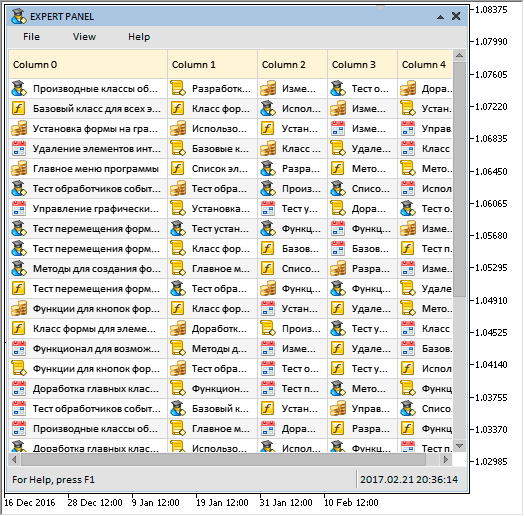Fig. 1. Table with icons in the cells.

### Highlighting the table rows when hovered

For the rows of the rendered table to be highlighted when hovered, additional fields and methods will be required. Use the CCanvasTable::LightsHover() method to enable the highlighting mode. The row color can be set with the help of the CCanvasTable::CellColorHover() method.

```class CCanvasTable : public CElement
{
private:
//--- Color of cells in different states
color             m_cell_color;
color             m_cell_color_hover;
//--- Mode of highlighting rows when hovered
bool              m_lights_hover;
//---
public:
//--- Color of cells in different states
void              CellColor(const color clr)           { m_cell_color=clr;                }
void              CellColorHover(const color clr)      { m_cell_color_hover=clr;          }
//--- Mode of highlighting rows when hovered
void              LightsHover(const bool flag)         { m_lights_hover=flag;             }
};
```

Highlighting a row does not require redrawing the entire table over and over as the cursor moves. Moreover, it is strongly recommended not to do so, because it greatly slows down the application and takes too many CPU resources. At the first/new entry of the mouse cursor to the area of the table, it is sufficient to look for the focus only once (iterate over the entire array of rows). The CCanvasTable::CheckRowFocus() method is used for this purpose. Once the focus is found and the row index is stored, simply check if the focus on the row with the stored index has changed when the cursor is moved. The described algorithm is implemented in the CCanvasTable::ChangeRowsColor() method, shown in the listing below. The CCanvasTable::RedrawRow() method is used for changing the row color, its code will be introduced later. The CCanvasTable::ChangeRowsColor() method is called in the CCanvasTable::ChangeObjectsColor() method to change the colors of the table objects.

```class CCanvasTable : public CElement
{
private:
//--- To determine the row focus
int               m_item_index_focus;
//--- To determine the moment of mouse cursor transition from one row to another
int               m_prev_item_index_focus;
//---
private:
//--- Changing the row color when hovered
void              ChangeRowsColor(void);
};
//+------------------------------------------------------------------+
//| Changing the row color when hovered                              |
//+------------------------------------------------------------------+
void CCanvasTable::ChangeRowsColor(void)
{
//--- Leave, if row highlighting when hovered is disabled
if(!m_lights_hover)
return;
//--- If not in focus
if(!m_table.MouseFocus())
{
//--- If not yet indicated that not in focus
if(m_prev_item_index_focus!=WRONG_VALUE)
{
m_item_index_focus=WRONG_VALUE;
//--- Change the color
RedrawRow();
m_table.Update();
//--- Reset the focus
m_prev_item_index_focus=WRONG_VALUE;
}
}
//--- If in focus
else
{
//--- Check the focus on the rows
if(m_item_index_focus==WRONG_VALUE)
{
//--- Get the index of the row with the focus
m_item_index_focus=CheckRowFocus();
//--- Change the row color
RedrawRow();
m_table.Update();
//--- Store as the previous index in focus
m_prev_item_index_focus=m_item_index_focus;
return;
}
//--- Get the relative Y coordinate below the mouse cursor
int y=m_mouse.RelativeY(m_table);
//--- Verifying the focus
bool condition=(y>m_rows[m_item_index_focus].m_y && y<=m_rows[m_item_index_focus].m_y2);
//--- If the focus changed
if(!condition)
{
//--- Get the index of the row with the focus
m_item_index_focus=CheckRowFocus();
//--- Change the row color
RedrawRow();
m_table.Update();
//--- Store as the previous index in focus
m_prev_item_index_focus=m_item_index_focus;
}
}
}
```

The CCanvasTable::RedrawRow() method for fast redrawing table rows operates in two modes:

•  when selecting a row
•  in the mode of highlighting a row when hovered.

The method needs to be passed the corresponding argument to specify the desired mode. By default, the argument is set to false, which indicates using the method in the mode of highlighting the table rows. The class contains special fields for both modes to determine the current and the previous selected/highlighted table row. Thus, marking another row requires redrawing only the previous and the current rows, and not the entire table.

The program leaves the method if the indexes are not defined (WRONG_VALUE). Next, it is necessary to determine how many indexes are defined. If this is the first entry to the table and only one index (current) is defined, then, accordingly, the color will be changed only in the current row. If entered again, the color will be changed in two rows (the current and the previous).

It is now necessary to determine the sequence for changing the row colors. If the index of the current row is greater than that of the previous, it means that the cursor moved down. Then, first change the color at the previous index, and then in the current one. In a reverse situation, do the opposite. The method also considers the moment of leaving the table area, when the index of the current row is not defined, while the index of the previous row is still present.

Once all the local variables for operation are initialized, the background of the rows, the grid, the icons and the text are drawn in strict sequence.

```class CCanvasTable : public CElement
{
private:
//--- Redraws the specified table row according to the specified mode
void              RedrawRow(const bool is_selected_row=false);
};
//+------------------------------------------------------------------+
//| Redraws the specified table row according to the specified mode  |
//+------------------------------------------------------------------+
void CCanvasTable::RedrawRow(const bool is_selected_row=false)
{
//--- The current and the previous row indexes
int item_index      =WRONG_VALUE;
int prev_item_index =WRONG_VALUE;
//--- Initialization of the row indexes relative to the specified mode
if(is_selected_row)
{
item_index      =m_selected_item;
prev_item_index =m_prev_selected_item;
}
else
{
item_index      =m_item_index_focus;
prev_item_index =m_prev_item_index_focus;
}
//--- Leave, if the indexes are not defined
if(prev_item_index==WRONG_VALUE && item_index==WRONG_VALUE)
return;
//--- The number of rows and columns for drawing
int rows_total    =(item_index!=WRONG_VALUE && prev_item_index!=WRONG_VALUE)? 2 : 1;
int columns_total =m_columns_total-1;
//--- Coordinates
int x1=1,x2=m_table_x_size;
int y1={0},y2={0};
//--- Array for values in a certain sequence
int indexes;
//--- If (1) the mouse cursor moved down or if (2) entering for the first time
if(item_index>m_prev_item_index_focus || item_index==WRONG_VALUE)
{
indexes=(item_index==WRONG_VALUE || prev_item_index!=WRONG_VALUE)? prev_item_index : item_index;
indexes=item_index;
}
//--- If the mouse cursor moved up
else
{
indexes=item_index;
indexes=prev_item_index;
}
//--- Draw the background of rows
for(int r=0; r<rows_total; r++)
{
//--- Calculate the coordinates of the upper and lower boundaries of the row
y1[r]=m_rows[indexes[r]].m_y+1;
y2[r]=m_rows[indexes[r]].m_y2-1;
//--- Determine the focus on the row with respect to the highlighting mode
bool is_item_focus=false;
if(!m_lights_hover)
is_item_focus=(indexes[r]==item_index && item_index!=WRONG_VALUE);
else
is_item_focus=(item_index==WRONG_VALUE)?(indexes[r]==prev_item_index) :(indexes[r]==item_index);
//--- Draw the row background
m_table.FillRectangle(x1,y1[r],x2,y2[r],RowColorCurrent(indexes[r],is_item_focus));
}
//--- Grid color
uint clr=::ColorToARGB(m_grid_color);
//--- Draw the borders
for(int r=0; r<rows_total; r++)
{
for(int c=0; c<columns_total; c++)
m_table.Line(m_columns[c].m_x2,y1[r],m_columns[c].m_x2,y2[r],clr);
}
//--- Draw the icons
for(int r=0; r<rows_total; r++)
{
for(int c=0; c<m_columns_total; c++)
{
//--- Draw the icon, if (1) it is present in this cell and (2) the text of this column is aligned to the left
if(ImagesTotal(c,r)>0 && m_columns[c].m_text_align==ALIGN_LEFT)
DrawImage(c,indexes[r]);
}
}
//--- To calculate the coordinates
int x=0,y=0;
//--- Text alignment mode
uint text_align=0;
//--- Draw the text
for(int c=0; c<m_columns_total; c++)
{
//--- Get (1) the X coordinate of the text and (2) the text alignment mode
x          =TextX(c);
text_align =TextAlign(c,TA_TOP);
//---
for(int r=0; r<rows_total; r++)
{
//--- (1) Calculate the coordinate and (2) draw the text
y=m_rows[indexes[r]].m_y+m_text_y_offset;
m_table.TextOut(x,y,m_columns[c].m_rows[indexes[r]].m_short_text,TextColor(c,indexes[r]),text_align);
}
}
}
```

This results in the following: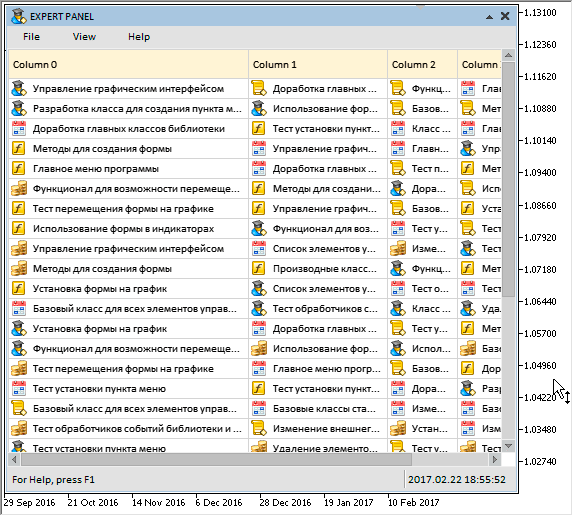Fig. 2. Demonstration of highlighting the table rows when hovered.

### Methods for fast redrawing the table cells

The methods for fast redrawing the table rows have been considered. The methods for fast redrawing the cells will be demonstrated now. For example, if it is necessary to change the text, its color or icon in any cell of the table, it is sufficient to redraw only the cell, and not the entire table. The private CCanvasTable::RedrawCell() method is used for this purpose. Only the cell content will be redrawn, while its frame will not be updated. The background color is determined by taking the highlighting mode into account, if enabled. After determining the values and initializing the local variables, the background, the icon (if assigned and if text is aligned to the left) and the text are drawn in the cell.

```class CCanvasTable : public CElement
{
private:
//--- Redraws the specified cell of the table
void              RedrawCell(const int column_index,const int row_index);
};
//+------------------------------------------------------------------+
//| Redraws the specified cell of the table                          |
//+------------------------------------------------------------------+
void CCanvasTable::RedrawCell(const int column_index,const int row_index)
{
//--- Coordinates
int x1=m_columns[column_index].m_x+1;
int x2=m_columns[column_index].m_x2-1;
int y1=m_rows[row_index].m_y+1;
int y2=m_rows[row_index].m_y2-1;
//--- To calculate the coordinates
int  x=0,y=0;
//--- To check the focus
bool is_row_focus=false;
//--- If the row highlighting mode is enabled
if(m_lights_hover)
{
//--- (1) Get the relative Y coordinate of the mouse cursor and (2) the focus on the specified table row
y=m_mouse.RelativeY(m_table);
is_row_focus=(y>m_rows[row_index].m_y && y<=m_rows[row_index].m_y2);
}
//--- Draw the cell background
m_table.FillRectangle(x1,y1,x2,y2,RowColorCurrent(row_index,is_row_focus));
//--- Draw the icon, if (1) it is present in this cell and (2) the text of this column is aligned to the left
if(ImagesTotal(column_index,row_index)>0 && m_columns[column_index].m_text_align==ALIGN_LEFT)
DrawImage(column_index,row_index);
//--- Get the text alignment mode
uint text_align=TextAlign(column_index,TA_TOP);
//--- Draw the text
for(int c=0; c<m_columns_total; c++)
{
//--- Get the X coordinate of the text
x=TextX(c);
//--- Stop the cycle
if(c==column_index)
break;
}
//--- (1) Calculate the Y coordinate, and (2) draw the text
y=y1+m_text_y_offset-1;
m_table.TextOut(x,y,m_columns[column_index].m_rows[row_index].m_short_text,TextColor(column_index,row_index),text_align);
}
```

Now let us consider the methods, which allow changing the text, the text color and the icon (selection from the assigned ones) in the cell. The public CCanvasTable::SetValue() and CCanvasTable::TextColor() methods must be used to set the text and its color. These methods are passed the indexes of the cell (column and row) and the value to be set. For the CCanvasTable::SetValue() method, it is a string value to be displayed in the cell. Here, the full passed string and its shortened version (if the full string does not fit the cell width) are stored to the corresponding fields of the table's structure (CTCell). The text color must be passed to the CCanvasTable::TextColor() method. As the fourth parameter in both methods, you can specify if it is necessary to redraw the cell immediately or it will be done later by calling the CCanvasTable::UpdateTable() method.

```class CCanvasTable : public CElement
{
private:
//--- Set the value to the specified table cell
void              SetValue(const uint column_index,const uint row_index,const string value,const bool redraw=false);
//--- Set the text color to the specified table cell
void              TextColor(const uint column_index,const uint row_index,const color clr,const bool redraw=false);
};
//+------------------------------------------------------------------+
//| Fills the array at the specified indexes                         |
//+------------------------------------------------------------------+
void CCanvasTable::SetValue(const uint column_index,const uint row_index,const string value,const bool redraw=false)
{
//--- Checking for exceeding the array range
if(!CheckOutOfRange(column_index,row_index))
return;
//--- Store the value into the array
m_columns[column_index].m_rows[row_index].m_full_text=value;
//--- Adjust and store the text, if it does not fit the cell
m_columns[column_index].m_rows[row_index].m_short_text=CorrectingText(column_index,row_index);
//--- Redraw the cell, if specified
if(redraw)
RedrawCell(column_index,row_index);
}
//+------------------------------------------------------------------+
//| Fill the text color array                                        |
//+------------------------------------------------------------------+
void CCanvasTable::TextColor(const uint column_index,const uint row_index,const color clr,const bool redraw=false)
{
//--- Checking for exceeding the array range
if(!CheckOutOfRange(column_index,row_index))
return;
//--- Store the text color in the common array
m_columns[column_index].m_rows[row_index].m_text_color=clr;
//--- Redraw the cell, if specified
if(redraw)
RedrawCell(column_index,row_index);
}
```

The icon in the cell can be changed by the CCanvasTable::ChangeImage() method. The index of the icon to switch to must be specified as the third parameter here. As in the previously described methods for changing the cell properties, it is possible to specify if the cell is to be redrawn immediately or later

```class CCanvasTable : public CElement
{
private:
//--- Changes the icon in the specified cell
void              ChangeImage(const uint column_index,const uint row_index,const uint image_index,const bool redraw=false);
};
//+------------------------------------------------------------------+
//| Changes the icon in the specified cell                           |
//+------------------------------------------------------------------+
void CCanvasTable::ChangeImage(const uint column_index,const uint row_index,const uint image_index,const bool redraw=false)
{
//--- Checking for exceeding the array range
if(!CheckOutOfRange(column_index,row_index))
return;
//--- Get the number of cell icons
int images_total=ImagesTotal(column_index,row_index);
//--- Leave, if (1) there are no icons or (2) out of range
if(images_total==WRONG_VALUE || image_index>=(uint)images_total)
return;
//--- Leave, if the specified icon matches the selected one
if(image_index==m_columns[column_index].m_rows[row_index].m_selected_image)
return;
//--- Store the index of the selected icon of the cell
m_columns[column_index].m_rows[row_index].m_selected_image=(int)image_index;
//--- Redraw the cell, if specified
if(redraw)
RedrawCell(column_index,row_index);
}
```

Another public method will be required to redraw the entire table — CCanvasTable::UpdateTable(). It can be called in two modes:

1. When it is necessary to simply update the table to display the recent changes made by the methods described above.
2. When it is necessary to completely redraw the table, if changes were made.

By default, the only argument of the method is set to false, which indicates updating without redrawing.

```class CCanvasTable : public CElement
{
private:
//--- Updating the table
void              UpdateTable(const bool redraw=false);
};
//+------------------------------------------------------------------+
//| Updating the table                                               |
//+------------------------------------------------------------------+
void CCanvasTable::UpdateTable(const bool redraw=false)
{
//--- Redraw the table, if specified
if(redraw)
DrawTable();
//--- Update the table
m_table.Update();
}
```

Below is the result of the work done: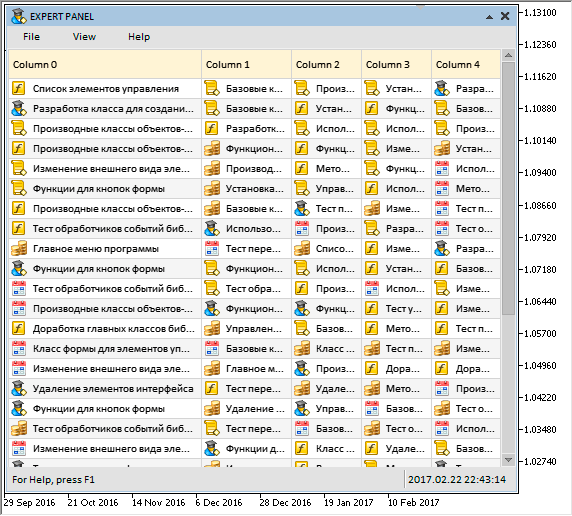Fig. 3. Demonstration of new features of the rendered table.

Expert Advisor with the demonstration of this result can be downloaded in the files attached to this article. During the program execution, the icons in all table cells (5 columns and 30 rows) will change at a frequency of 100 milliseconds. The screenshot below shows the CPU load without the user interaction with the graphical interface of the MQL application. CPU load with the update frequency of 100 milliseconds does not exceed 3%.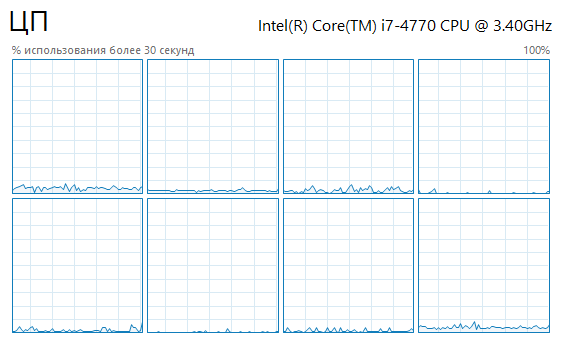Fig. 4. The CPU load during the execution of the test MQL application.

### Application for testing the controls

The current version of the rendered table is already "smart" enough to create the same tables as in the Market Watch window, for instance. Let us try to demonstrate this. For the example, create a table of 5 columns and 25 rows. Those will be the 25 symbols available on the MetaQuotes-Demo server. The data in the table will be the following:

• Symbol – financial instruments (currency pairs).
• Bid – Bid prices.
• Ask – Ask prices.
• Spread (!) – difference between the Bid and Ask prices.
• Time – the time of the last quote.

Let us prepare the same icons for denoting the latest changes in the price as in the table of the Market Watch window. The first initialization of the table cells will be done immediately in the method of creating the control and it will be performed by calling the auxiliary CProgram :: InitializingTable() method of the custom class.

```//+------------------------------------------------------------------+
//| Class for creating the application                               |
//+------------------------------------------------------------------+
class CProgram : public CWndEvents
{
private:
//--- Initialize the table
void              InitializingTable(void);
};
//+------------------------------------------------------------------+
//| Initialize the table                                             |
//+------------------------------------------------------------------+
void CProgram::InitializingTable(void)
{
//--- Array of the header titles
string text_headers[COLUMNS1_TOTAL]={"Symbol","Bid","Ask","!","Time"};
//--- Array of symbols
string text_array=
{
"AUDUSD","GBPUSD","EURUSD","USDCAD","USDCHF","USDJPY","NZDUSD","USDSEK","USDHKD","USDMXN",
"USDZAR","USDTRY","GBPAUD","AUDCAD","CADCHF","EURAUD","GBPCHF","GBPJPY","NZDJPY","AUDJPY",
"EURJPY","EURCHF","EURGBP","AUDCHF","CHFJPY"
};
//--- Array of icons
string image_array=
{
"::Images\\EasyAndFastGUI\\Icons\\bmp16\\circle_gray.bmp",
"::Images\\EasyAndFastGUI\\Icons\\bmp16\\arrow_up.bmp",
"::Images\\EasyAndFastGUI\\Icons\\bmp16\\arrow_down.bmp"
};
//---
for(int c=0; c<COLUMNS1_TOTAL; c++)
{
//--- Set the header titles
m_canvas_table.SetHeaderText(c,text_headers[c]);
//---
for(int r=0; r<ROWS1_TOTAL; r++)
{
//--- Set the icons
m_canvas_table.SetImages(c,r,image_array);
//--- Set the symbol names
if(c<1)
m_canvas_table.SetValue(c,r,text_array[r]);
//--- Default value for all cells
else
m_canvas_table.SetValue(c,r,"-");
}
}
}
```

The values of these table cells will be updated by timer every 16 milliseconds during the runtime. Another auxiliary CProgram::UpdateTable() method has been created for this purpose. Here, the program leaves the method if it is a day of the weekend (Saturday or Sunday). Then, a double loop iterates over all columns and rows of the table. This double loop obtains the last two ticks for each symbol and after the changes in prices are analyzed, sets the corresponding values.

```class CProgram : public CWndEvents
{
private:
//--- Initialize the table
void              InitializingTable(void);
};
//+------------------------------------------------------------------+
//| Updating the table values                                        |
//+------------------------------------------------------------------+
void CProgram::UpdateTable(void)
{
MqlDateTime check_time;
::TimeToStruct(::TimeTradeServer(),check_time);
//--- Leave, if it is a Saturday or Sunday
if(check_time.day_of_week==0 || check_time.day_of_week==6)
return;
//---
for(int c=0; c<m_canvas_table.ColumnsTotal(); c++)
{
for(int r=0; r<m_canvas_table.RowsTotal(); r++)
{
//--- The symbol to get the data for
string symbol=m_canvas_table.GetValue(0,r);
//--- Get the data of the last two ticks
MqlTick ticks[];
if(::CopyTicks(symbol,ticks,COPY_TICKS_ALL,0,2)<2)
continue;
//--- Set the array as time series
::ArraySetAsSeries(ticks,true);
//--- Column of symbols - Symbol. Determine the price direction.
if(c==0)
{
int index=0;
//--- If the prices did not change
if(ticks.ask==ticks.ask && ticks.bid==ticks.bid)
index=0;
//--- If the Bid price changed upward
else if(ticks.bid>ticks.bid)
index=1;
//--- If the Bid price changed downward
else if(ticks.bid<ticks.bid)
index=2;
//--- Set the corresponding icon
m_canvas_table.ChangeImage(c,r,index,true);
}
else
{
//--- Column of the price difference - Spread (!)
if(c==3)
{
//--- Get and set the spread size in points
int spread=(int)::SymbolInfoInteger(symbol,SYMBOL_SPREAD);
m_canvas_table.SetValue(c,r,string(spread),true);
continue;
}
//--- Get the number of decimal places
int digit=(int)::SymbolInfoInteger(symbol,SYMBOL_DIGITS);
//--- Column of the Bid prices
if(c==1)
{
m_canvas_table.SetValue(c,r,::DoubleToString(ticks.bid,digit));
//--- If the price changed, set the color corresponding to the direction
if(ticks.bid!=ticks.bid)
m_canvas_table.TextColor(c,r,(ticks.bid<ticks.bid)? clrRed : clrBlue,true);
//---
continue;
}
//--- Column of the Ask prices
if(c==2)
{
m_canvas_table.SetValue(c,r,::DoubleToString(ticks.ask,digit));
//--- If the price changed, set the color corresponding to the direction
if(ticks.ask!=ticks.ask)
m_canvas_table.TextColor(c,r,(ticks.ask<ticks.ask)? clrRed : clrBlue,true);
//---
continue;
}
//--- Column of the last arrival time of the symbol prices
if(c==4)
{
long   time     =::SymbolInfoInteger(symbol,SYMBOL_TIME);
string time_msc =::IntegerToString(ticks.time_msc);
int    length   =::StringLen(time_msc);
string msc      =::StringSubstr(time_msc,length-3,3);
string str      =::TimeToString(time,TIME_MINUTES|TIME_SECONDS)+"."+msc;
//---
color clr=clrBlack;
//--- If the prices did not change
if(ticks.ask==ticks.ask && ticks.bid==ticks.bid)
clr=clrBlack;
//--- If the Bid price changed upward
else if(ticks.bid>ticks.bid)
clr=clrBlue;
//--- If the Bid price changed downward
else if(ticks.bid<ticks.bid)
clr=clrRed;
//--- Set the value and text color
m_canvas_table.SetValue(c,r,str);
m_canvas_table.TextColor(c,r,clr,true);
continue;
}
}
}
}
//--- Update the table
m_canvas_table.UpdateTable();
}
```

The following result is obtained: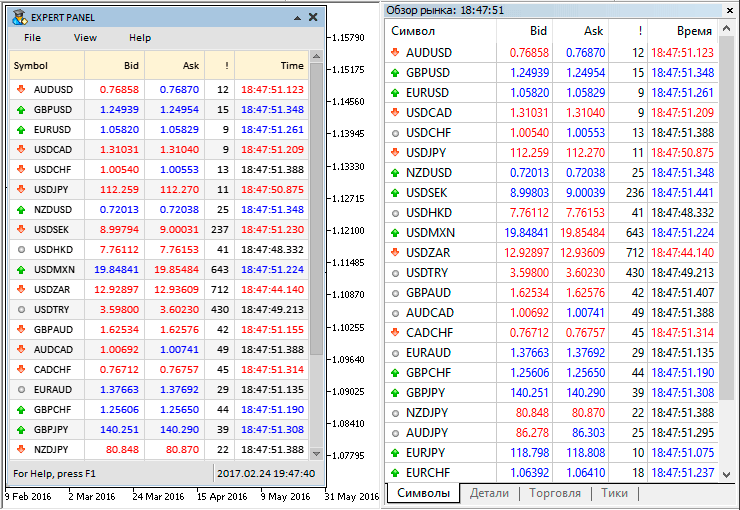Fig. 5. Comparison of the data in the Market Watch window and the custom analog.

The test application featured in the article can be downloaded using the below link for further studying.

### Conclusion

The library for creating graphical interfaces at the current stage of development looks like in the schematic below.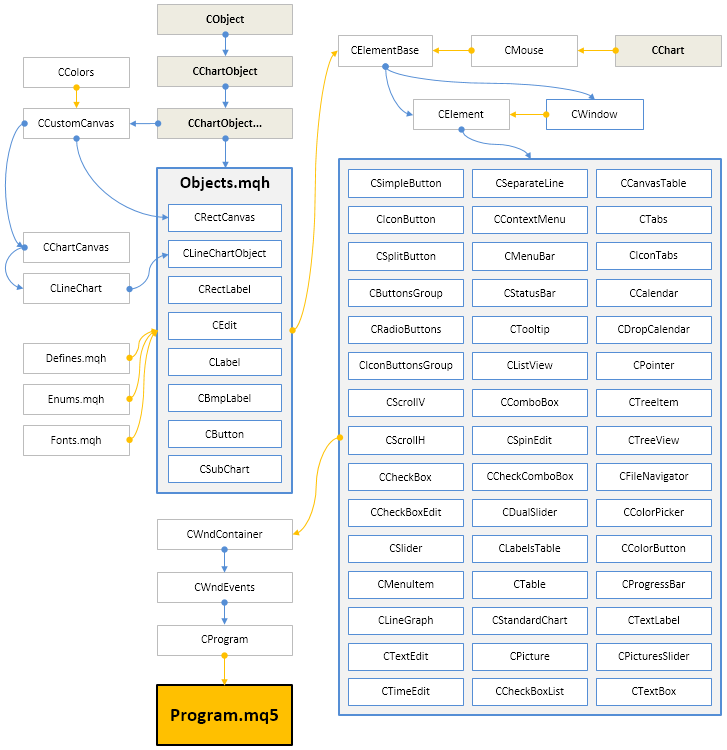Fig. 6. Structure of the library at the current stage of development.

Below you can download the latest version of the library and files for testing.

If you have questions on using the material presented in those files, you can refer to the detailed description of the library development in one of the articles of this series or ask your question in the comments of this article.

Translated from Russian by MetaQuotes Software Corp.
Original article: https://www.mql5.com/ru/articles/3042

Attached files |

#### Other articles by this author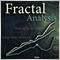Calculating the Hurst exponent
The article thoroughly explains the idea behind the Hurst exponent, as well as the meaning of its values and the calculation algorithm. A number of financial market segments are analyzed and the method of working with MetaTrader 5 products implementing the fractal analysis is described.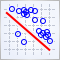Visualize this! MQL5 graphics library similar to 'plot' of R language
When studying trading logic, visual representation in the form of graphs is of great importance. A number of programming languages popular among the scientific community (such as R and Python) feature the special 'plot' function used for visualization. It allows drawing lines, point distributions and histograms to visualize patterns. In MQL5, you can do the same using the CGraphics class.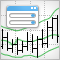A Universal Channel with the Graphical Interface
All channel indicators are displayed as three lines, including central, top and bottom lines. The drawing principle of the central line is similar to a moving average, while the moving average indicator is mostly used for drawing channels. The top and bottom lines are located at equal distances from the central line. This distance can be determined in points, as percent of price (the Envelopes indicator), using a standard deviation value (Bollinger Bands), or an ATR value (Keltner channel).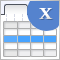Graphical interfaces X: New features for the Rendered table (build 9)
Until today, the CTable was the most advanced type of tables among all presented in the library. This table is assembled from edit boxes of the OBJ_EDIT type, and its further development becomes problematic. Therefore, in terms of maximum capabilities, it is better to develop rendered tables of the CCanvasTable type even at the current development stage of the library. Its current version is completely lifeless, but starting from this article, we will try to fix the situation.